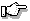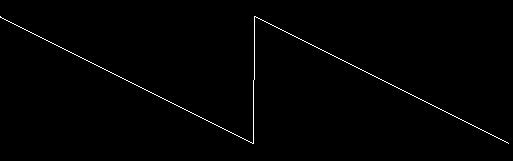# PgDrawTrend(), PgDrawTrendmx()

Draw a trend graph

## Synopsis:

```int PgDrawTrend( short const *ptr,
PhPoint_t const *pos,
int num,
int delta,
int buflen,
int bufoff,
unsigned flags );

int PgDrawTrendmx( short const *ptr,
PhPoint_t const *pos,
int num,
int delta,
int buflen,
int bufoff,
unsigned flags );```

## Description:

These functions build a command in the draw buffer to draw a trend graph.

The ptr argument points to an array of short values. Each of these values is used as either the x or the y coordinate; the other coordinate is calculated by adding delta to the previous value.

For example, if flags is Pg_TREND_VERT, the coordinates would be calculated as follows:

```pos.x + *(ptr+0), pos.y + (delta * 0)
pos.x + *(ptr+1), pos.y + (delta * 1)
pos.x + *(ptr+2), pos.y + (delta * 2)
...
pos.x + *(ptr+num-1), pos.y + (delta * (num-1))```

The pos argument defines the origin of the trend graph and the num argument controls the number of values to be drawn.

The flags argument controls how the trend will be drawn. It must be one of the following:

Pg_TREND_HORIZ
Draw a horizontal graph. The ptr values become the y axis and the delta values are added to the x coordinate.
Pg_TREND_VERT
Draw a vertical graph. The ptr values become the x axis and the delta values are added to the y coordinate.

The buflen and bufoff arguments aren't currently used, and must be set to 0 for future compatibility.If you call the "mx" form of this function, the data isn't physically copied into the draw buffer. Instead, a pointer to the array is stored until the draw buffer is flushed. Make sure you call PgFlush() before you modify the array. If the data is in shared memory, the mx form of this function will automatically pass a shared memory reference instead of the array.

## Returns:

0
Success.
-1
The draw buffer is too small to hold the current draw state, the draw command and the data. Increase the size of the draw buffer, or decrease the number of points.

## Examples:

The following example:

```void HTrend() {
short    data;
PhPoint_t    p = { 0, 80 };
int i;

for (i=0; i<512; i++) data[i] = (i & 127) - 64;
PgSetStrokeColor( Pg_WHITE );
PgDrawTrend( &data, &p, 256, 2, 0, 0,
Pg_TREND_HORIZ );

}```

will draw:## Classification:

Photon

Safety:
Interrupt handler No
Signal handler No
Thread No